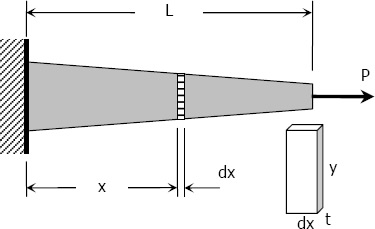# Axial Deformation

In the linear portion of the stress-strain diagram, the tress is proportional to strain and is given by

$\sigma = E \varepsilon$

since $\sigma = P / A$ and $\varepsilon = \delta / L$, then $\dfrac{P}{A} = E \dfrac{\delta}{L}$

$\delta = \dfrac{PL}{AE} = \dfrac{\sigma L}{E}$

To use this formula, the load must be axial, the bar must have a uniform cross-sectional area, and the stress must not exceed the proportional limit.

If however, the cross-sectional area is not uniform, the axial deformation can be determined by considering a differential length and applying integration.$\delta = \displaystyle \dfrac{P}{E} \int_0^L \frac{dx}{L}$

where A = ty, and y and t if variable, must be expressed in terms of x.

For a rod of unit mass ρ suspended vertically from one end, the total elongation due to its own weight is

$\delta = \dfrac{\rho g L^2}{2E} = \dfrac{MgL}{2AE}$

where ρ is in kg/m3, L is the length of the rod in mm, M is the total mass of the rod in kg, A is the cross-sectional area of the rod in mm2, and g = 9.81 m/s2.

Stiffness, k
Stiffness is the ratio of the steady force acting on an elastic body to the resulting displacement. It has the unit of N/mm.

$k = \dfrac{P}{\delta}$